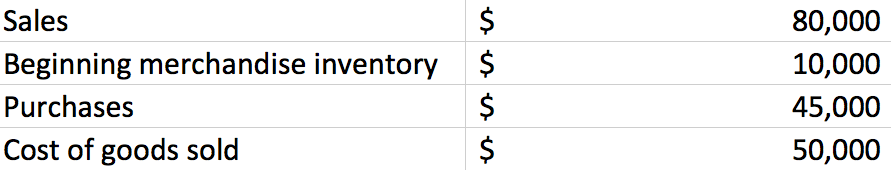# Problem: Consider the following: Eighty-three units were sold. What is the value assigned to the ending inventory using the FIFO method of inventory costing? a. $5,000 b.$30,000 c. $35,000 d.$10,000

###### Problem Details

Consider the following:

Eighty-three units were sold. What is the value assigned to the ending inventory using the FIFO method of inventory costing?

a. $5,000 b.$30,000

c. $35,000 d.$10,000Frequently Asked Questions

What scientific concept do you need to know in order to solve this problem?

Our tutors have indicated that to solve this problem you will need to apply the Periodic Inventory - FIFO, LIFO, and Average Cost concept. You can view video lessons to learn Periodic Inventory - FIFO, LIFO, and Average Cost. Or if you need more Periodic Inventory - FIFO, LIFO, and Average Cost practice, you can also practice Periodic Inventory - FIFO, LIFO, and Average Cost practice problems.

What is the difficulty of this problem?

Our tutors rated the difficulty ofConsider the following: Eighty-three units were sold. What ...as low difficulty.